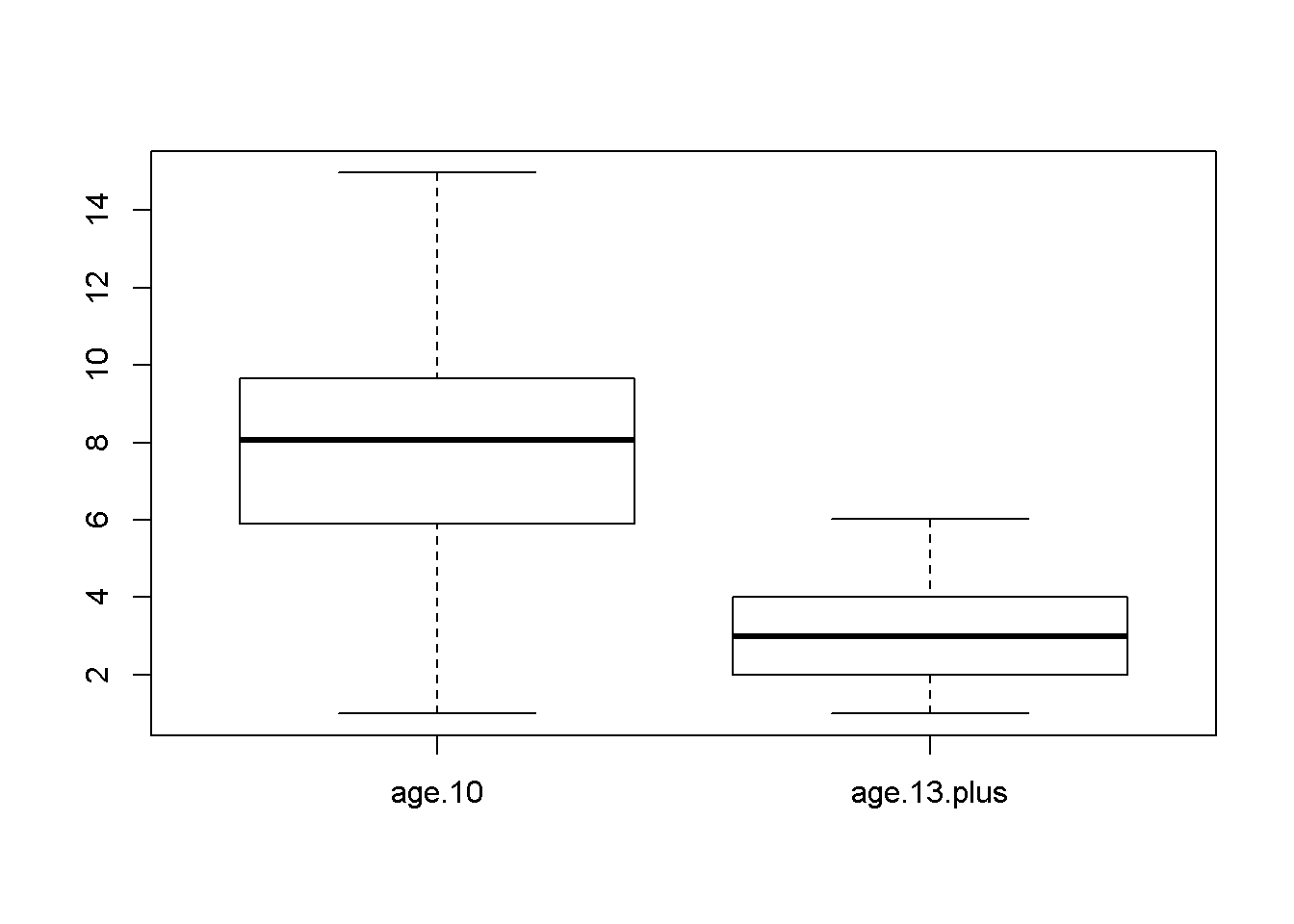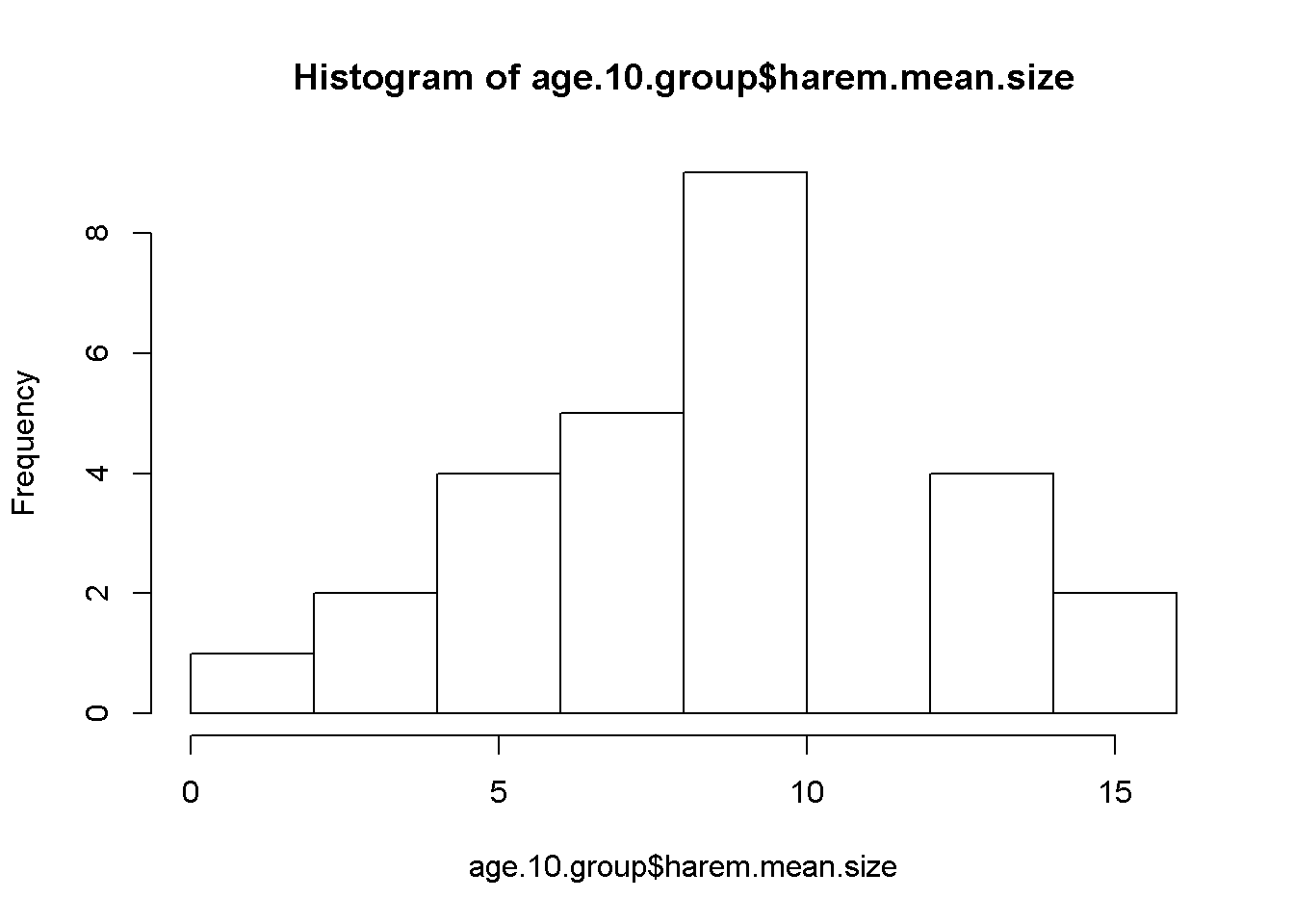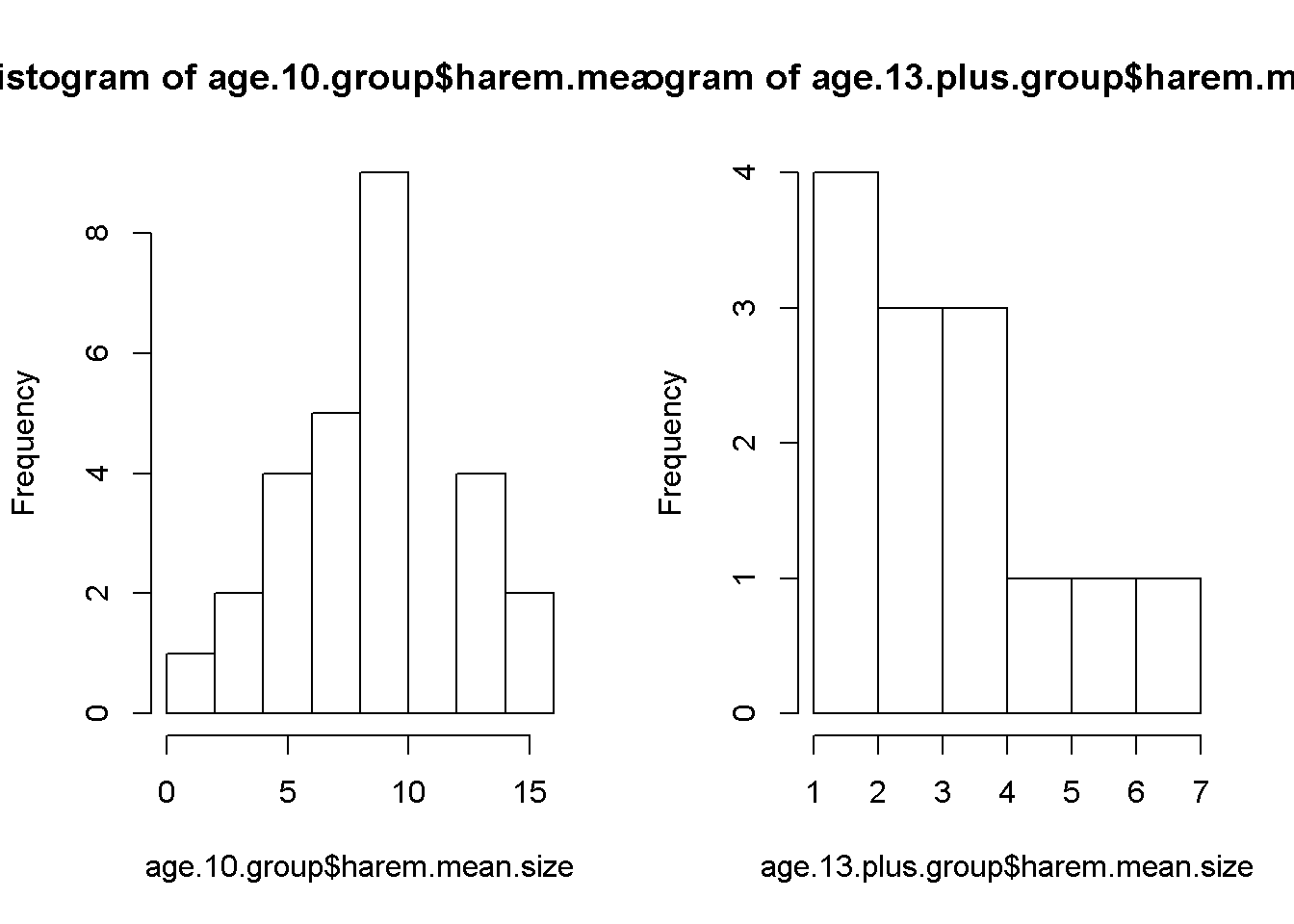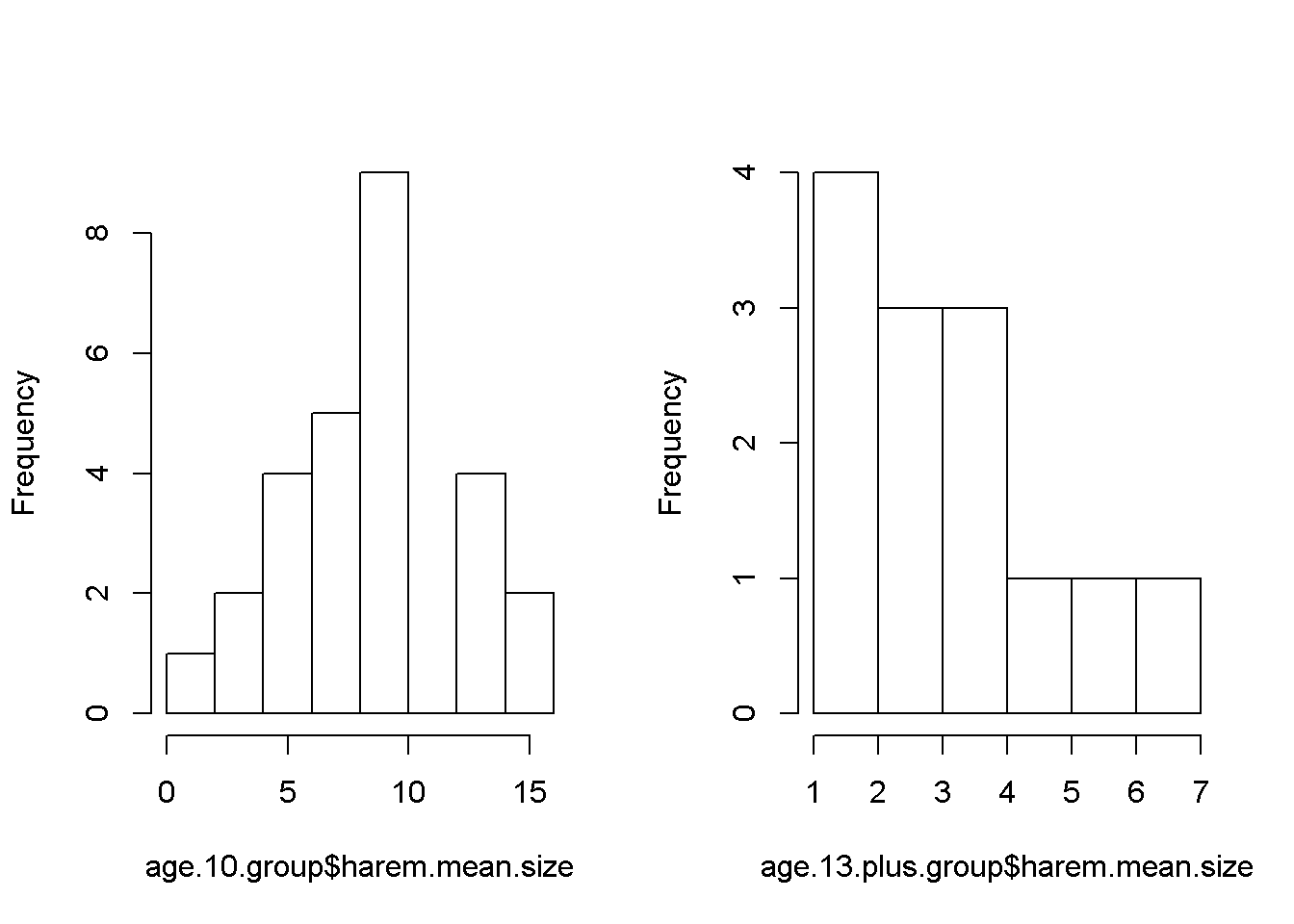Working with grouped data

This lab shows how to work with a dataset where we want to summarize the information by two separate groups. In this case, its male red deer of two different ages, 10 years only and 13+ years old.

Preliminaries

Set the appropriate working directly

setwd("C:/Users/lisanjie2/Desktop/TEACHING/1_STATS_CalU/1_STAT_CalU_2016_by_NLB/Lab/Lab4b")

Summary statistics w/ summary()

If a variable is numeric (continous)

• mean
• median
• interquartile range

If a variable is categorical (factor)

• sample size in each category
summary(harem)
##        age.class  harem.mean.size
##  age.10     :27   Min.   : 1.000
##  age.13.plus:13   1st Qu.: 3.524
##                   Median : 6.346
##                   Mean   : 6.615
##                   3rd Qu.: 8.712
##                   Max.   :14.963

Note that the summary command DOES NOT split the data up by the categorical variable. The mean, median etc reported is for the entire column of data. It is therefore not very meaningful.

Boxplot with grouped data

When data is organized by groups in a spreadsheet R can automatically split it up by group and make boxplot. The boxplot() function splits up the two groups automatically. Other functions that can do this include t.test() for t tests and lm() for regression and ANOVA. Not all basic R functions, however, deal with grouped data so easily.

boxplot(harem.mean.size ~ age.class, data = harem)Using subset() to split up a dataframe

The subset() command can split data up. It takes on several arguments.

• “x = harem”" designates the dataframe we are working with
• “select = c(…)” designates the columns in the datafrme we are interested in
• “age.class ==”age.10" defines a “logical condition” by which we want to split the data

Note that he only thing in qutoes is “age.10”, and that age.class is follwed by TWO equals signs.

age.10.group <- subset(x = harem,
select = c(age.class, harem.mean.size),
age.class == "age.10")

In words, this function read “subset the harem data set for me; grab the age.class and harem.mean.size columns”, and give me back just the rows of data where age.class equals “age.10”.

To get the “age.13.plus” subset of data we change the “age.class =…” part of this code

age.13.plus.group <- subset(x = harem,
select = c(age.class, harem.mean.size),
age.class == "age.13.plus")

We now split our origina harem dataframe into two separate, new data frames. We can use the summary command on each one separately

summary(age.10.group)
##        age.class  harem.mean.size
##  age.10     :27   Min.   : 1.000
##  age.13.plus: 0   1st Qu.: 5.894
##                   Median : 8.071
##                   Mean   : 8.220
##                   3rd Qu.: 9.642
##                   Max.   :14.963
summary(age.13.plus.group)
##        age.class  harem.mean.size
##  age.10     : 0   Min.   :1.000
##  age.13.plus:13   1st Qu.:2.000
##                   Median :3.000
##                   Mean   :3.284
##                   3rd Qu.:4.000
##                   Max.   :6.037

Plotting two histograms

The hist() does not have any automatic feature for spiltting up data. We can make histograms for each subgroup by calling hist() on each one separately

For the age 10 group

hist(age.10.group$harem.mean.size)For the age 13+ group hist(age.10.group$harem.mean.size)Notice that we use a very different grammer for this command than the boxplot command; there is no “~”. There is a“$”. The way this command reads is “make a histogram of the age.10.group dataframe, using the column for harem.mean.size”. Plotting 2 plots next to each other using par() We can plot these two histograms next to each other, but it requires a function in R that is bit obscure, par, and an arguement in it mfrow. What this will do is change the plotting parameters, and tells it R to make 2 plots next to each other. First, the par() command par(mfrow = c(1,2)) Notice that when we run this command nothing happens. Now, make a histogram. Run both hist command back to back par(mfrow = c(1,2)) hist(age.10.group$harem.mean.size)
hist(age.13.plus.group$harem.mean.size)The text that gets put automatically at the top of the graph is kinda annoying. We can get rid of it using the arguement main = “” . The “” means “nothing” par(mfrow = c(1,2)) hist(age.10.group$harem.mean.size, main = "")
hist(age.13.plus.group$harem.mean.size, main = "")Getting summary statistics by group using tapply() The tapply() function can split data up into groups and then apply a function to the groups, giving you just a summary of the data. This is really handy for calculating and then plotting the means of two groups. First, we’ll calcualte the mean of the data in the harem$$harem.mean.size column, splitting up by the groups found in harem$$age.class, using the mean function. tapply(harem$harem.mean.size,  #the numeric variable
harem$age.class, #the categorical variable FUN = mean, #the mathematical operation na.rm = T) ## age.10 age.13.plus ## 8.219715 3.283557 We can store that information in an object. my.means <- tapply(harem$harem.mean.size,  #the numeric variable
harem$age.class, #the categorical variable FUN = mean, #the mathematical operation na.rm = T) my.means ## age.10 age.13.plus ## 8.219715 3.283557 We can do this for other functions, such as the standard deviation. my.sd <- tapply(harem$harem.mean.size,  #the numeric variable
harem$age.class, #the categorical variable FUN = sd, #the mathematical operation na.rm = T) my.sd ## age.10 age.13.plus ## 3.519256 1.645208 Using the length() function, we can get the sample size of each group. my.sample.size <- tapply(harem$harem.mean.size,  #the numeric variable
harem\$age.class,        #the categorical variable
FUN = length
)

my.sample.size
##      age.10 age.13.plus
##          27          13

We can then do math on these objects. Here, we can take both sd values and divide them by the square root of the sample size to get the standard error. Note that R allows you to do math on sets of numbers and it keeps them straigth for you.

my.se <- my.sd/sqrt(my.sample.size)